# New preprint: Allocating police resources while limiting racial inequality

I have a new working paper out, Allocating police resources while limiting racial inequality. In this work I tackle the problem that a hot spots policing strategy likely exacerbates disproportionate minority contact (DMC). This is because of the pretty simple fact that hot spots of crime tend to be in disadvantaged/minority neighborhoods.

Here is a graph illustrating the problem. X axis is the proportion of minorities stopped by the police in 500 by 500 meter grid cells (NYPD data). Y axis is the number of violent crimes over along time period (12 years). So a typical hot spots strategy would choose the top N areas to target (here I do top 20). These are all very high proportion minority areas. So the inevitable extra police contact in those hot spots (in the form of either stops or arrests) will increase DMC.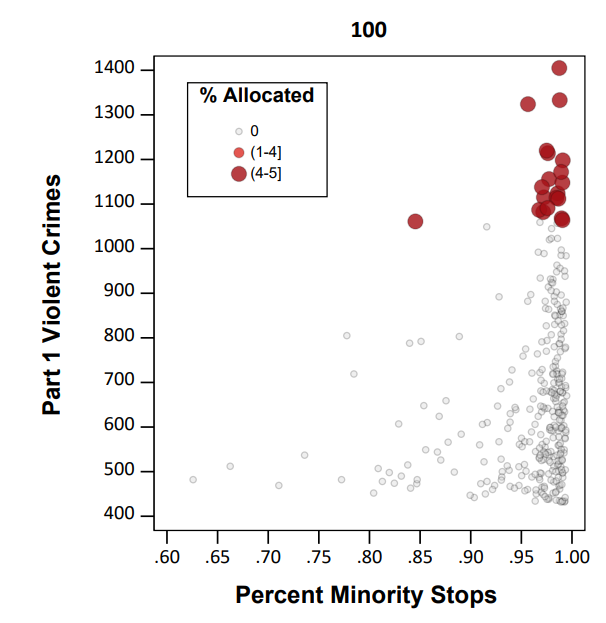I’d note that the majority of critiques of predictive policing focus on whether reported crime data is biased or not. I think that is a bit of a red herring though, you could use totally objective crime data (say swap out acoustic gun shot sensors with reported crime) and you still have the same problem.

The proportion of stops by the NYPD of minorities has consistently hovered around 90%, so doing a bunch of extra stuff in those hot spots will increase DMC, as those 20 hot spots tend to have 95%+ stops of minorities (with the exception of one location). Also note this 90% has not changed even with the dramatic decrease in stops overall by the NYPD.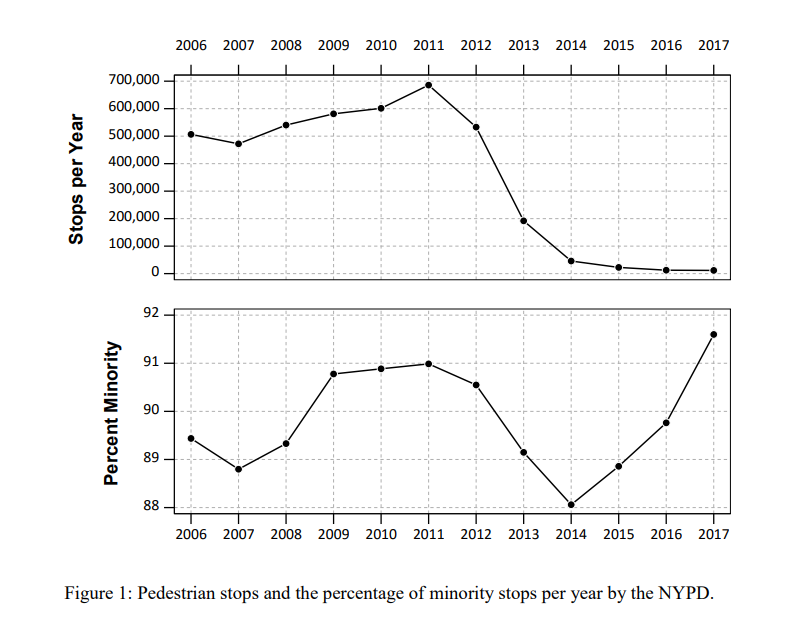So to illustrate my suggested solution here is a simple example. Consider you have a hot spot with predicted 30 crimes vs a hot spot with predicted 28 crimes. Also imagine that the 30 crime hot spot results in around 90% stops of minorities, whereas the 28 crime hot spot only results in around 50% stops of minorities. If you agree reducing DMC is a reasonable goal for the police in-and-of-itself, you may say choosing the 28 crime area is a good idea, even though it is a less efficient choice than the 30 crime hot spot.

I show in the paper how to codify this trade-off into a linear program that says choose X hot spots, but has a constraint based on the expected number of minorities likely to be stopped. Here is an example graph that shows it doesn’t always choose the highest crime areas to meet that racial equity constraint.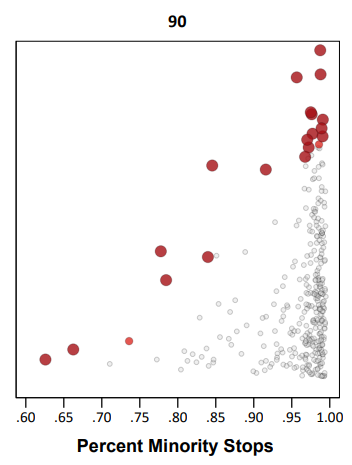This results in a trade-off of efficiency though. Going back to the original hypothetical, trading off a 28 crime vs 30 crime area is not a big deal. But if the trade off was 3 crimes vs 30 that is a bigger deal. In this example I show that getting to 80% stops of minorities (NYC is around 70% minorities) results in hot spots with around 55% of the crime compared to the no constraint hot spots. So in the hypothetical it would go from 30 crimes to 17 crimes.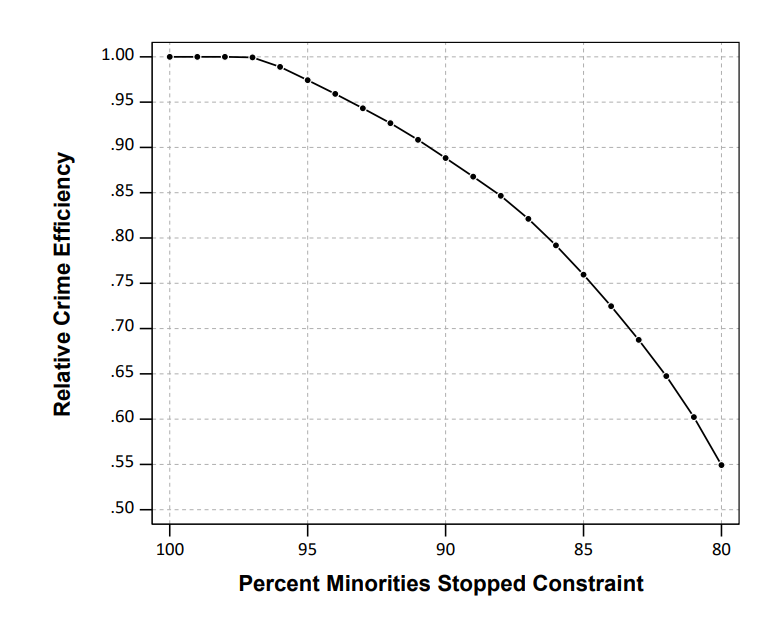There won’t be a uniform formula to calculate the expected decrease in efficiency, but I think getting to perfect equality with the residential pop. will typically result in similar large decreases in many scenarios. A recent paper by George Mohler and company showed similar fairly steep declines. (That uses a totally different method, but I think will be pretty similar outputs in practice — can tune the penalty factor in a similar way to changing the linear program constraint I think.)

So basically the trade-off to get perfect equity will be steep, but I think the best case scenario is that a PD can say "this predictive policing strategy will not make current levels of DMC worse" by applying this algorithm on-top-of your predictive policing forecasts.

I will be presenting this work at ASC, so stop on by! Feedback always appreciated.

# New preprint: The accuracy of the violent offender identification directive (VOID) tool to predict future gun violence

I have a new preprint out, The accuracy of the violent offender identification directive (VOID) tool to predict future gun violence. This is work with Rob Worden and Jasmine Silver from our time at the Finn Institute. Below is the abstract:

We evaluate the Violent Offender Identification Directive (VOID) tool, a risk assessment instrument implemented within a police department to prospectively identify offenders likely to be involved with future gun violence. The tool uses a variety of static measures of prior criminal history that are readily available in police records management systems. The VOID tool is assessed for predictive accuracy by taking a historical sample and calculating scores for over 200,000 individuals known to the police at the end of 2012, and predicting 103 individuals involved with gun violence (either as a shooter or a victim) during 2013. Despite weights for the instrument being determined in an ad-hoc manner by crime analysts, the VOID tool does very well in predicting involvement with gun violence compared to an optimized logistic regression and generalized boosted models. We discuss theoretical reasons why such ad-hoc instruments are likely to perform well in identifying chronic offenders for all police departments.

There were just slightly over 100 violent gun offenders we were trying to pick out of over 200,000. The VOID tool did really well! Here is a graph comparing how many of those offenders VOID captured compared to a generalized boosted model (GBM), and two different logistic regression equations.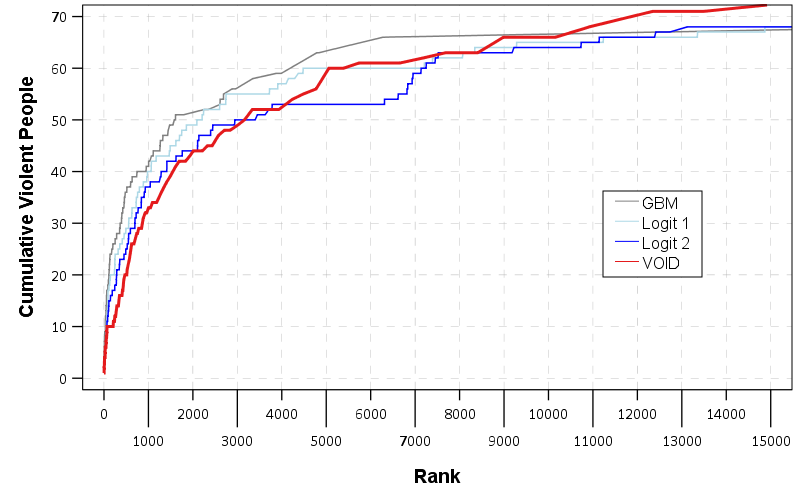I have some of my thoughts in this article as to why a simple tool does just as well as more complicated regression and machine learning techniques, which is a common finding in recidivism studies as well. My elevator pitch for why that is is because most offenders are generalists, and for example you can basically swap prior arrests for robbery with prior arrests for motor vehicle theft — they both provide essentially the same signal for future potential criminality. See also discussion of this on Dan Simpson’s post on the Stat Modeling, Causal Inference and Social Science blog, which in turn makes me think the idea behind simple models can be readily applied to many decision points in the criminal justice field.

The simple takeaway from this for crime analysts making chronic offender lists is that don’t let the perfect be the enemy of the good. Analysts can likely create an ad-hoc weighting to prioritize chronic offenders and it will do quite well compared to fancier models.

I will be presenting this work at the ACJS conference in New Orleans on Saturday 2/17/18. It is a great session, with YongJei Lee, Jerry Ratcliffe, Bryanna Fox, and Stacy Sechrist (see session 384 in the ACJS program), so stop on by. If you want to catch up with me in New Orleans just send me an email. And as always if you have feedback on the draft I am all ears.

# How wide to make the net in actuarial tools? (false positives versus false negatives)

An interesting debate/question came up in my work recently. I conducted an analysis of a violence risk assessment tool for a police department. Currently the PD takes around the top 1,000 scores of this tool, and then uses further intelligence and clinical judgements to place a small number of people on a chronic offender list (who are then subject to further interventions). My assessment of the predictive validity when examining ROC curves suggested the tool does a pretty good job discriminating violent people up to around the top 6,000 individuals and after that flattens out. In a sample of over 200,000, the top 1000 scores correctly classified 30 of the 100 violent cases, and the top 6000 classified 60.

So the question came up should we recommend that the analysts widen the net to the top 6,000 scores, instead of only examining the top 1,000 scores? There are of course costs and limitations of what the analysts can do. It may simply be infeasible for the analysts to review 6,000 people. But how do you set the limit? Should the clinical assessments be focused on even fewer individuals than 1,000?

We can make some estimates of where the line should be drawn by setting weights for the cost of a false positive versus a false negative. Implicit in the whole exercise of predicting violence in a small set of people is that false negatives (failing to predict someone will be violent when they are) greatly outweigh a false positive (predicting someone will be violent but they are not). The nature of the task dictates that you will always need to have quite a few false positives to classify even a few true positives, and no matter what you do there will only be a small number of false negatives.

Abstractly, you can place a value on the cost of failing to predict violence, and a cost on the analysts time to evaluate cases. In this situation we want to know whether the costs of widening the net to 6,000 individuals are less than the costs of only examining the top 1,000 individuals. Here I will show we don’t even need to know what the exact cost of a false positive or a false negative is, only the relative costs, to make an estimate about whether the net should be cast wider.

The set up is that if we only take the top 1,000 scores, it will capture 30 out of the 100 violent cases. So there will be (100 – 30) false negatives, and (1000 – 30) false positives. If we increase the scores to evaluate the top 6,000, it will capture 60 out the 100 violent cases, but then we will have (6000 – 60) false positives. I can not assign a specific number to the cost of a false negative and a false positive. So we can write these cost equations as:

``````1) (100 - 30)*FN + (1000 - 30)*FP = Cost Low
2) (100 - 60)*FN + (6000 - 60)*FP = Cost High``````

Even though we do not know the exact cost of a false negative, we can talk about relative costs, e.g. `1 false negative = 1000*false positives`. There are too many unknowns here, so I am going to set `FP = 1`. This makes the numbers relative, not absolute. So with this constraint the reduced equations can be written as:

``````1) 70*FN +  970 = Cost Low
2) 40*FN + 5940 = Cost High``````

So we want to know the ratio at which there is a net benefit over including the top 6,000 scores versus only the top 1,000. So this means that `Cost High < Cost Low`. To figure out this point, we can subtract equation 2 from equation 1:

``3) (70 - 40)*FN - 4970 = Cost Low - Cost High``

If we set this equation to zero and solve for FN we can find the point where these two equations are equal:

``````30*FN - 4970 = 0
30*FN = 4970
FN = 4970/30 = 165 + 2/3``````

If the value of a false negative is more than 166 times the value of a false positive, `Cost Low - Cost High` will be positive, and so the false negatives are more costly to society relative to the analysts time spent. It is still hard to make guesses as to whether the cost of violence to society is 166 times more costly than the analysts time, but that is at least one number to wrap your head around. In a more concrete example, such as granting parole or continuing to be incarcerated, given how expensive prison is net widening (with these example numbers) would probably not be worth it. But here it is a bit more fuzzy especially because the analysts time is relatively inexpensive. (You also have to guess how well you can intervene, in the prison example incarceration essentially reduces the probability of committing violence to zero, whereas police interventions can not hope to be that successful.)

As long as you assume that the classification rate is linear within this range of scores, the same argument holds for net widening any number. But in reality there are diminishing returns the more scores you examine (and 6,000 is basically where the returns are near zero). If you conduct the same exercise between classifying zero and the top 1,000, the rate of the cost of a false negative to a false positive needs be `32+1/3` to justify evaluating the top 1,000 scores. If you actually had an estimate of the ratio of the cost of false positives to false negatives you could then figure out exactly how wide to make the net. But if you think the ratio is well above 166, you have plenty of reason to widen the net to the larger value.

# ROC and Precision-Recall curves in SPSS

Recently I was tasked with evaluating a tool used to predict violence. I initially created some code to plot ROC curves in SPSS for multiple classifiers, but then discovered that the `ROC` command did everything I wanted. Some recommend precision-recall curves in place of ROC curves, especially when the positive class is rare. This fit my situation (a few more than 100 positive cases in a dataset of 1/2 million) and it was pretty simple to adapt the code to return the precision. I will not go into the details of the curves (I am really a neophyte at this prediction stuff), but here are a few resources I found useful:

The macro is named `!Roc` and it takes three parameters:

• `Class` – the numeric classifier (where higher equals a greater probability of being predicted)
• `Target` – the outcome you are trying to predict. Positive cases need to equal 1 and negative cases 0
• `Suf` – this the the suffix on the variables returned. The procedure returns “Sens[Suf]”, “Spec[Suf]” and “Prec[Suf]” (which are the sensitivity, specificity, and precision respectively).

So here is a brief made up example using the macro to draw ROC and precision and recall curves (entire syntax including the macro can be found here). So first lets make some fake data and classifiers. Here `Out` is the target being predicted, and I have two classifiers, `X` and `R`. `R` is intentionally made to be basically random. The last two lines show an example of calling the macro.

``````SET SEED 10.
INPUT PROGRAM.
LOOP #i = 20 TO 70.
COMPUTE X = #i + RV.UNIFORM(-10,10).
COMPUTE R = RV.NORMAL(45,10).
COMPUTE Out = RV.BERNOULLI(#i/100).
END CASE.
END LOOP.
END FILE.
END INPUT PROGRAM.
DATASET NAME RocTest.
DATASET ACTIVATE RocTest.
EXECUTE.

!Roc Class = X Target = Out Suf = "_X".
!Roc Class = R Target = Out Suf = "_R".``````

Now we can make an ROC curve plot with this information. Here I use inline `TRANS` statements to calculate 1 minus the specificity. I also use a blending trick in GPL to make the beginning of the lines connect at (0,0) and the end at (1,1).

``````*Now make a plot with both classifiers on it.
GGRAPH
/GRAPHDATASET NAME="graphdataset" VARIABLES=Spec_X Sens_X Spec_R Sens_R
/GRAPHSPEC SOURCE=INLINE.
BEGIN GPL
PAGE: begin(scale(770px,600px))
SOURCE: s=userSource(id("graphdataset"))
DATA: Spec_X=col(source(s), name("Spec_X"))
DATA: Sens_X=col(source(s), name("Sens_X"))
DATA: Spec_R=col(source(s), name("Spec_R"))
DATA: Sens_R=col(source(s), name("Sens_R"))
TRANS: o = eval(0)
TRANS: e = eval(1)
TRANS: SpecM_X = eval(1 - Spec_X)
TRANS: SpecM_R = eval(1 - Spec_R)
COORD: rect(dim(1,2), sameRatio())
GUIDE: axis(dim(1), label("1 - Specificity"), delta(0.1))
GUIDE: axis(dim(2), label("Sensitivity"), delta(0.1))
GUIDE: text.title(label("ROC Curve"))
SCALE: linear(dim(1), min(0), max(1))
SCALE: linear(dim(2), min(0), max(1))
ELEMENT: edge(position((o*o)+(e*e)), color(color.lightgrey))
ELEMENT: line(position(smooth.step.right((o*o)+(SpecM_R*Sens_R)+(e*e))), color("R"))
ELEMENT: line(position(smooth.step.right((o*o)+(SpecM_X*Sens_X)+(e*e))), color("X"))
PAGE: end()
END GPL.``````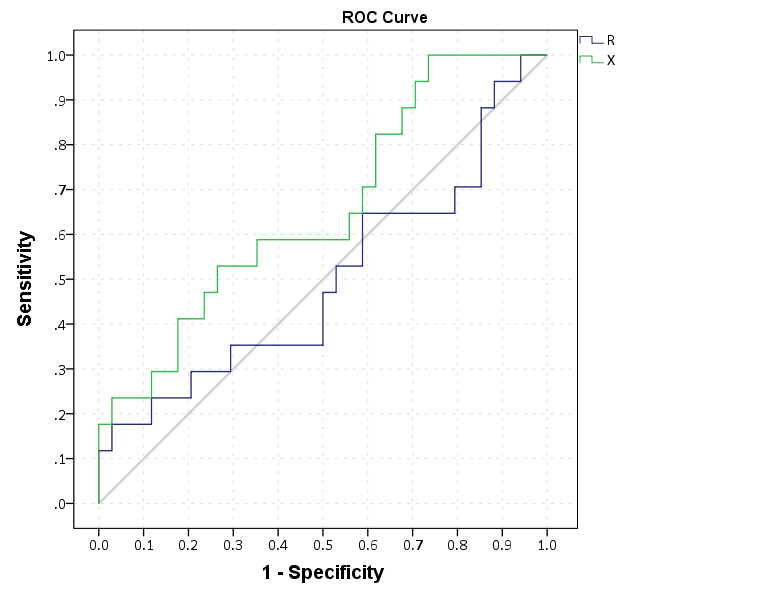This just replicates the native SPSS `ROC` command though, and that command returns other useful information as well (such as the actual area under the curve). We can see though that my calculations of the curve are correct.

``````*Compare to SPSS's ROC command.
ROC R X BY Out (1)
/PLOT CURVE(REFERENCE)
/PRINT SE COORDINATES.``````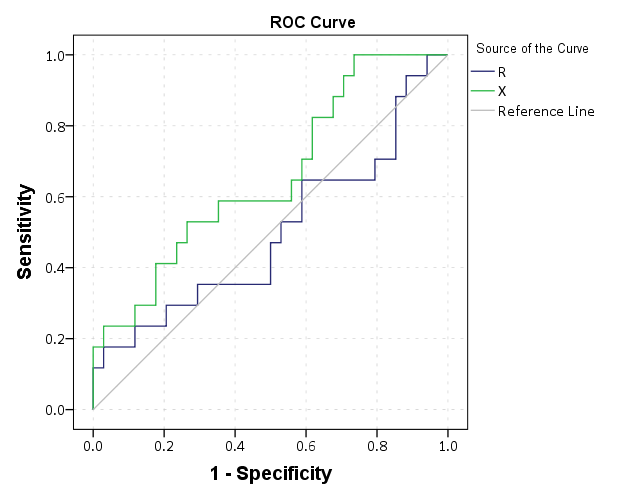To make a precision-recall graph we need to use the `path` element and sort the data in a particular way. (SPSS’s `line` element works basically the opposite of the way we need it to produce the correct sawtooth pattern.) The blending trick does not work with this graph, but it is immaterial in interpreting the graph.

``````*Now make precision recall curves.
*To make these plots, need to reshape and sort correctly, so the path follows correctly.
VARSTOCASES
/MAKE Sens FROM Sens_R Sens_X
/MAKE Prec FROM Prec_R Prec_X
/MAKE Spec FROM Spec_R Spec_X
/INDEX Type.
VALUE LABELS Type
1 'R'
2 'X'.
SORT CASES BY Sens (A) Prec (D).
GGRAPH
/GRAPHDATASET NAME="graphdataset" VARIABLES=Sens Prec Type
/GRAPHSPEC SOURCE=INLINE.
BEGIN GPL
PAGE: begin(scale(770px,600px))
SOURCE: s=userSource(id("graphdataset"))
DATA: Sens=col(source(s), name("Sens"))
DATA: Prec=col(source(s), name("Prec"))
DATA: Type=col(source(s), name("Type"), unit.category())
COORD: rect(dim(1,2), sameRatio())
GUIDE: axis(dim(1), label("Recall"), delta(0.1))
GUIDE: axis(dim(2), label("Precision"), delta(0.1))
GUIDE: text.title(label("Precision-Recall Curve"))
SCALE: linear(dim(1), min(0), max(1))
SCALE: linear(dim(2), min(0), max(1))
ELEMENT: path(position(Sens*Prec), color(Type))
PAGE: end()
END GPL.
*The sawtooth is typical.``````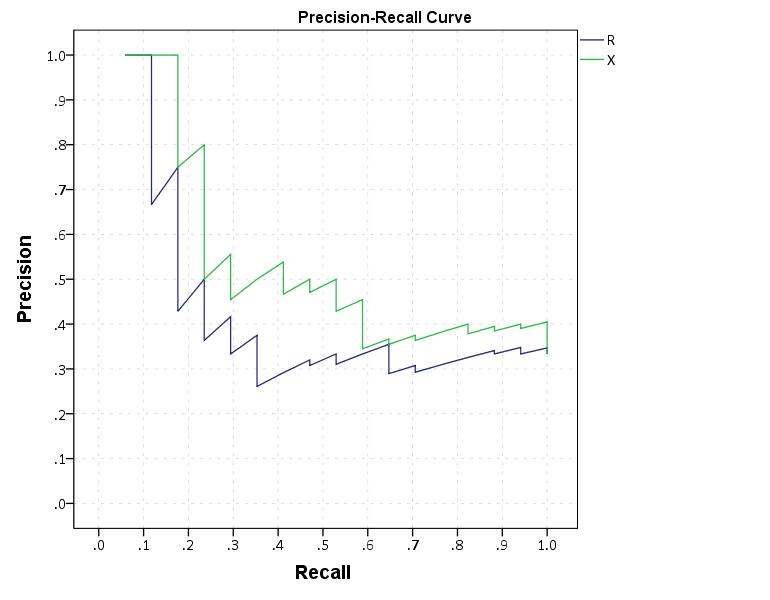These curves both show that `X` is the clear winner. In my use application the ROC curves are basically superimposed, but there is more separation in the precision-recall graph. Being very generic, most of the action in the ROC curve is at the leftmost area of the graph (with only a few positive cases), but the PR curve is better at identifying how wide you have to cast the net to find the few positive cases. In a nut-shell, you have to be willing to live with many false positives to be able to predict just the few positive cases.

I would be interested to hear other analysts perspective. Predicting violence is a popular topic in criminology, with models of varying complexity. But what I’m finding so far in this particular evaluation is basically that there are set of low hanging fruit of chronic offenders that score high no matter how much you crunch the numbers (around 60% of the people who committed serious violence in a particular year in my sample), and then a set of individuals with basically no prior history (around 20% in my sample). So basically ad-hoc scores are doing about as well predicting violence as more complicated machine learning models (even machine learning models fit on the same data).